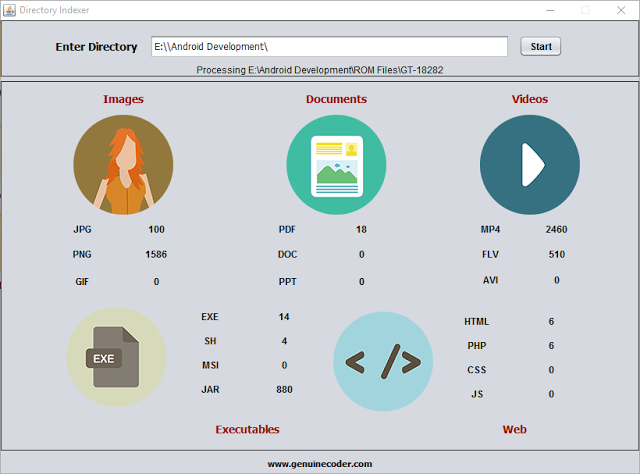# Directory / Folder Indexing Program Using JavaI thought it will be cool if I can make a program that scans some pen drive or hard disk connected to my PC in order find out how many images,videos or documents are there. In simple terms, a directory or folder/file indexing program. Thanks to java again.
Directory indexer uses the FILE class from java.io package. An object of FILE can store both files and directories. The parent or Root directory, from where we start indexing is read from the user using normal JTextField. Then a function called processDirectory() is called with argument as the given input text.
The function, processDirectory() then create a new File with argument as given path. Then the list of files and directories present inside the directory are taken as a file array using listFiles() method from File class. If you are interested in finding more about file listing, read How tocreate a file explorer in java.
`` File[] listFiles = fl.listFiles();  ``
After getting the list of contents in the given directory, a for loop is applied which will go through all the available contents (objects of File class). As we said earlier, it can be either a file or a directory. If it is a file, then we check its extension by splitting the name string using “.” Symbol. The fun thing about extension is, a file can contain more than 1 dotes. For example, I want to name my file as “genuine.coder.image.jpg”. It is perfectly alright to use this filename and if we go for splitting and taking the string after first dot, we will get “.coder” which is definitely not what we are expecting. So the solution is to find the string after last dot and it is achieved using the code
``````String[] extension = listFile.getName().split("[.]");
String ex = extension[extension.length - 1]; ``````
So now we have the extension. All that we have to do is to check the string against the required extension (jpg,png,pdf etc) and increment the counter.
`````` if (ex.equalsIgnoreCase("jpg")) {
jpg.setText(Integer.parseInt(jpg.getText()) + 1 + "");
}  ``````
I found this way of incrementing the counter as a simple one (not efficient at all) since I don’t have to maintain some arrays for each separate extensions.
If the file object which is being processed is not a file, then it may be a directory. If it is, then we have to get inside of that and index them also. A simple recursive call to the function will do the trick.
`````` if (listFile.isDirectory()) {
processDirectory(listFile.getAbsolutePath()); //Recursive call to processDirectoy
}  ``````
I have used a thread to call the processDirectory() function. If I don’t, the updating on the UI (incrementing the counter) will be lagging. Using just one new thread, it is possible to achieve better refreshing and processing rates. For this purpose, a new class named directoryProcessor is created and implemented as Runnable which is then passed as a runnable object for new Thread object. The processDirectory is called from inside the run() method of directoryProcessor.
Class directoryProcessor
``````class directoryProcessor implements Runnable {
@Override
public void run() {
processDirectory(input.getText());
sts.setText("Completed");
JOptionPane.showMessageDialog(rootPane, "Indexing Completed", "Done", JOptionPane.INFORMATION_MESSAGE);
}
}  ``````
Function processDirectory()

``````public void processDirectory(String dir) {
File fl = new File(dir);
sts.setText("Processing " + dir);
File[] listFiles = fl.listFiles();
for (File listFile : listFiles) {
if (listFile.isFile()) {
String[] extension = listFile.getName().split("[.]");
String ex = (extension[extension.length - 1]);
if (ex.equalsIgnoreCase("jpg")) {
jpg.setText(Integer.parseInt(jpg.getText()) + 1 + "");
} else if (ex.equalsIgnoreCase("png")) {
png.setText(Integer.parseInt(png.getText()) + 1 + "");
} else if (ex.equalsIgnoreCase("gif")) {
gif.setText(Integer.parseInt(gif.getText()) + 1 + "");
} else if (ex.equalsIgnoreCase("ppt")) {
ppt.setText(Integer.parseInt(ppt.getText()) + 1 + "");
} else if (ex.equalsIgnoreCase("doc")) {
doc.setText(Integer.parseInt(doc.getText()) + 1 + "");
} else if (ex.equalsIgnoreCase("pdf")) {
pdf.setText(Integer.parseInt(pdf.getText()) + 1 + "");
} else if (ex.equalsIgnoreCase("mp4")) {
mp4.setText(Integer.parseInt(mp4.getText()) + 1 + "");
} else if (ex.equalsIgnoreCase("flv")) {
flv.setText(Integer.parseInt(flv.getText()) + 1 + "");
} else if (ex.equalsIgnoreCase("avi")) {
avi.setText(Integer.parseInt(avi.getText()) + 1 + "");
} else if (ex.equalsIgnoreCase("exe")) {
exe.setText(Integer.parseInt(exe.getText()) + 1 + "");
} else if (ex.equalsIgnoreCase("sh")) {
sh.setText(Integer.parseInt(sh.getText()) + 1 + "");
} else if (ex.equalsIgnoreCase("jar")) {
jar.setText(Integer.parseInt(jar.getText()) + 1 + "");
} else if (ex.equalsIgnoreCase("html")) {
html.setText(Integer.parseInt(html.getText()) + 1 + "");
} else if (ex.equalsIgnoreCase("php")) {
php.setText(Integer.parseInt(php.getText()) + 1 + "");
} else if (ex.equalsIgnoreCase("css")) {
css.setText(Integer.parseInt(css.getText()) + 1 + "");
} else if (ex.equalsIgnoreCase("js")) {
js.setText(Integer.parseInt(js.getText()) + 1 + "");
}
} else if (listFile.isDirectory()) {
try {
processDirectory(listFile.getAbsolutePath());
} catch (Exception e) {
System.err.println("Exception - " + e.getMessage());
}
}
}
} ``````Muhammed Afsal Villan is an experienced full-stack developer, specialized in desktop and mobile application development. He also regularly publishes quality tutorials on his YouTube channel named 'Genuine Coder'. He likes to contribute to open-source projects and is always enthusiastic about new technologies.